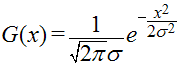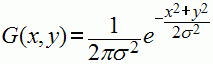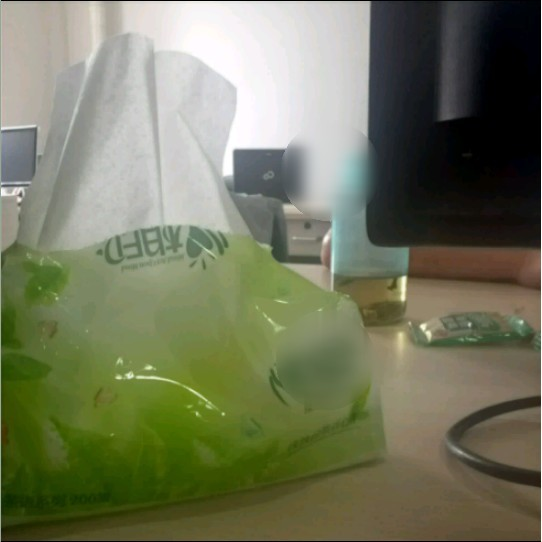# 高斯模糊算法（gaussian）

2012/07/04 11:51

- 理论 -或者一个二维的函数G(x,y)G(0) = 0.3989422804

G(1) = G(-1) = 0.2419707245

G(2) = G(-2) = 0.0539909665

G(3) = G(-3) = 0.0044318484

......

......

- 实际应用 -

1、模糊后的画面，其发光度和亮度大都比原始图像要低，画面显得很暗
2、上述的采样点（tap）的标准差是随意的吗？或者说，它有什么要求（或限制）？

ΣHBlur = G(0) + G(-1) + G(1) + G(-2) + G(2)

ΣHBlur = 0.9908656625

AugFactor = 1 / ΣHBlur

AugFactor = 1.009218543

σ = 1 -> G(2)aug = 0.054488685

σ = 1.745 -> G(3)aug = 0.054441945

σ = 2.7 -> G(4)aug = 0.054409483

- 一维复合运算 | 二维单一运算 -

1、使用一维函数的两次;一次横向，然后（以横向模糊的结果）再做一次垂直运算 ；

2、用二维函数，只需要一步就能完成。

/**
* 高斯模糊算法
*
* @Author : Lei Guoting
* @Date   : 2012-07-04
*
* @Param inPixels 原始图片Pixels
* @Param outPixels 处理后输出图片Pixels
* @Circle 自定义结构体，定义一个需要被高斯模糊处理的园的位置大小
* @AndroidBitmapInfo 原始图片Bitmap信息
*/
void gaussian(void** inPixels,void** outPixels,Circle tGassCir,AndroidBitmapInfo bitmapInfo)
{
uint32_t x,y;
uint32_t tx,ty;
uint8_t *tInPixel, *tOutPixel;
double tR = 0.0,tG = 0.0,tB = 0.0,tCount = 0.0;
double ar = 8,dif,gauss;
int range = ar * 3;
int startx,endx,starty,endy;
int m;
int sx,sy;
uint32_t top,left,right,bottom;
LOGD("filter @gaussian center.x=%d,center.y=%d",tGassCir.center.x,tGassCir.center.y);
top = tGassCir.center.y - tGassCir.r;
bottom = tGassCir.center.y + tGassCir.r;
left = tGassCir.center.x - tGassCir.r;
right = tGassCir.center.x + tGassCir.r;
if(top < 0){
top = 0;
}
if(bottom > bitmapInfo.height - 1){
bottom = bitmapInfo.height - 1;
}
if(left < 0){
left = 0;
}
if(right > bitmapInfo.width - 1){
right = bitmapInfo.width - 1;
}
//The dimension of X
LOGD("filter @gaussian begin..");
LOGD("top=%d,bottom=%d,left=%d,right=%d",top,bottom,left,right);
Point tDo[(bottom - top +1) * (right - left +1)];
uint32_t tDoLen = 0;
for(y = top; y <= bottom; y ++){
for(x = left; x <= right; x ++){
/*
if(sqrt(pow((x - tGassCir.center.x),2) + pow((y - tGassCir.center.y),2)) > tGassCir.r){
continue;
}
*/

if(sqrt((x - tGassCir.center.x) * (x - tGassCir.center.x) + (y - tGassCir.center.y) * (y - tGassCir.center.y)) > tGassCir.r){
continue;
}
Point p = {x,y};
tDo[tDoLen] = p;
tDoLen ++;

tOutPixel = (uint8_t* )*outPixels + (y * bitmapInfo.width + x) * 4;
startx = (x < range ? -x : -range);
endx = ((bitmapInfo.width <= x + range) ? (bitmapInfo.width - x - 1) : range);

//x dimension
tR = tG = tB = tCount = 0.0;
for(m = startx; m <= endx; m ++){
tInPixel = (uint8_t* )*inPixels + (y * bitmapInfo.width + (x + m)) * 4;
dif = m*m;
gauss = exp(-dif/(2*ar*ar));
tR += gauss * *(tInPixel + 0);
tG += gauss * *(tInPixel + 1);
tB += gauss * *(tInPixel + 2);
tCount += gauss;
}
*(tOutPixel + 0) = tR / tCount;
*(tOutPixel + 1) = tG / tCount;
*(tOutPixel + 2) = tB / tCount;
}
}

//y dimension
uint32_t ind = 0;
Point p;
for(ind = 0; ind < tDoLen; ind ++){
p = tDo[ind];
tOutPixel = (uint8_t* )*outPixels + (p.y * bitmapInfo.width + p.x) * 4;
starty = (p.y < range ? -p.y : -range);
endy = ((bitmapInfo.height <= p.y + range) ? (bitmapInfo.height - p.y - 1) : range);
tR = tG = tB = tCount = 0.0;
for(m = starty; m <= endy; m ++){
tInPixel = (uint8_t* )*outPixels + ((p.y + m) * bitmapInfo.width + p.x) * 4;
dif = m*m;
gauss = exp(-dif/(2*ar*ar));
tR += gauss * *(tInPixel + 0);
tG += gauss * *(tInPixel + 1);
tB += gauss * *(tInPixel + 2);
tCount += gauss;
}
*(tOutPixel + 0) = tR / tCount;
*(tOutPixel + 1) = tG / tCount;
*(tOutPixel + 2) = tB / tCount;
}
LOGD("filter @gaussian end!");
}

typedef struct{
uint32_t x;
uint32_t y;
}Point;

typedef struct{
Point center;
int r;
}Circle;

gaussian加权的时候为了尽量快一点时间，稍微省略了点。### 作者的其它热门文章

2
4 收藏

3 评论
4 收藏
2